# Cars and Trucks

Kurt has seven shelves. Kurt puts three cars on each odd number shelf. Kurt puts four trucks on each even number shelf. Does Kurt have the same total number of cars and trucks on the even shelves as he does on the odd number shelves? Show all your mathematical thinking.

Assessment

## Plan

#### Formal Mathematical Language and Symbolic Notation

A student may independently select a printed number line, number chart, ten frames, graph paper, etc. as they work on a task.

### Suggested materials

Engagement Image:

Teachers may project the image below to launch this task for their students, define nouns, promote discussion, access prior knowledge, and inspire engagement and problem solving.

## Solutions tabs

### Expert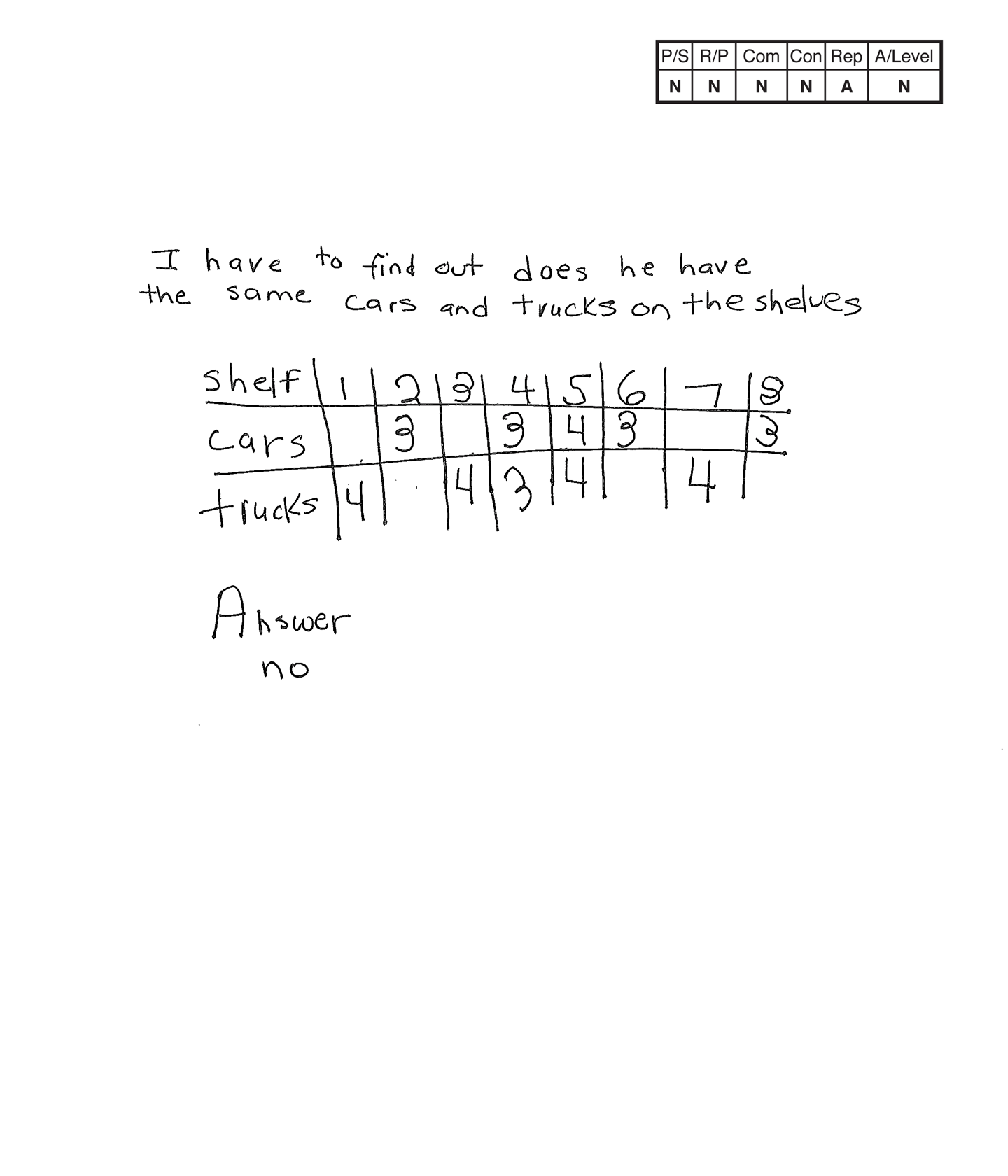This student does not meet the standard.

### Scoring Rationale

#### Novice

The student's table indicating eight shelves, with cars and trucks on some of the same shelves does not work to solve the task. The student's answer, "no," is not correct.

#### Novice

The student does not show correct reasoning of the underlying concepts of the task. The student does not consistently place three cars on each odd-number shelf and four trucks on each even-number shelf to determine that there are an equal number of twelve cars and trucks on the shelves.

#### Novice

The student does not use any mathematical language to communicate their reasoning and proof.

#### Novice

The student does not make a mathematically relevant observation about their solution.

#### Apprentice

The student's table is appropriate to the task but is not accurate. There are only seven shelves and cars should only be on the odd-numbered shelves and trucks on the even-numbered shelves.

#### Novice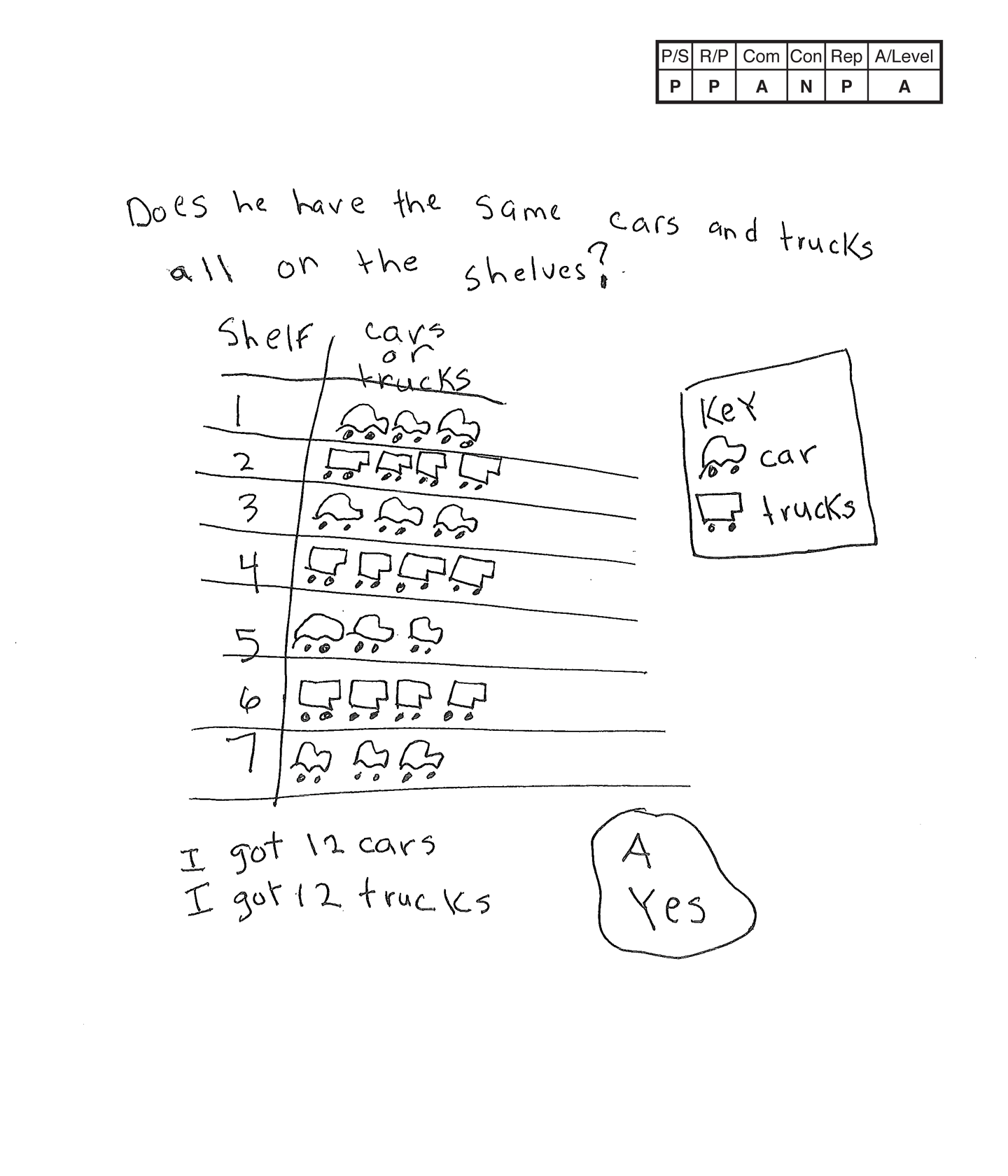This student does not meet the standard.

### Scoring Rationale

#### Practitioner

The student's table indicating seven shelves with three cars on each odd shelf and four trucks on each even shelf for a total of twelve cars and twelve trucks works to solve the task. The student's answer, "Yes," is correct.

#### Practitioner

The student shows correct reasoning of the underlying concepts of the task. The student understands odd, even, three cars to one shelf, four trucks to one shelf, and comparing two totals.

#### Apprentice

The student correctly uses the mathematical term key.

#### Novice

The student does not make a mathematically relevant observation about their solution.

#### Practitioner

The student's table is appropriate to the problem and accurate. A key defines the cars and trucks, each column is labeled correctly and all entered data is correct.

#### Apprentice

Note:

The overall achievement level for this piece of student work falls under Exemplars exception to the rule category. If a student has all Apprentice scores or above, but a Novice in "Connections," the student may still receive an achievement level score of Apprentice. To learn more about Exemplars scoring, please refer to the section of your dashboard called "Tools for Success" and click on the link for “Using the Assessment Rubric.”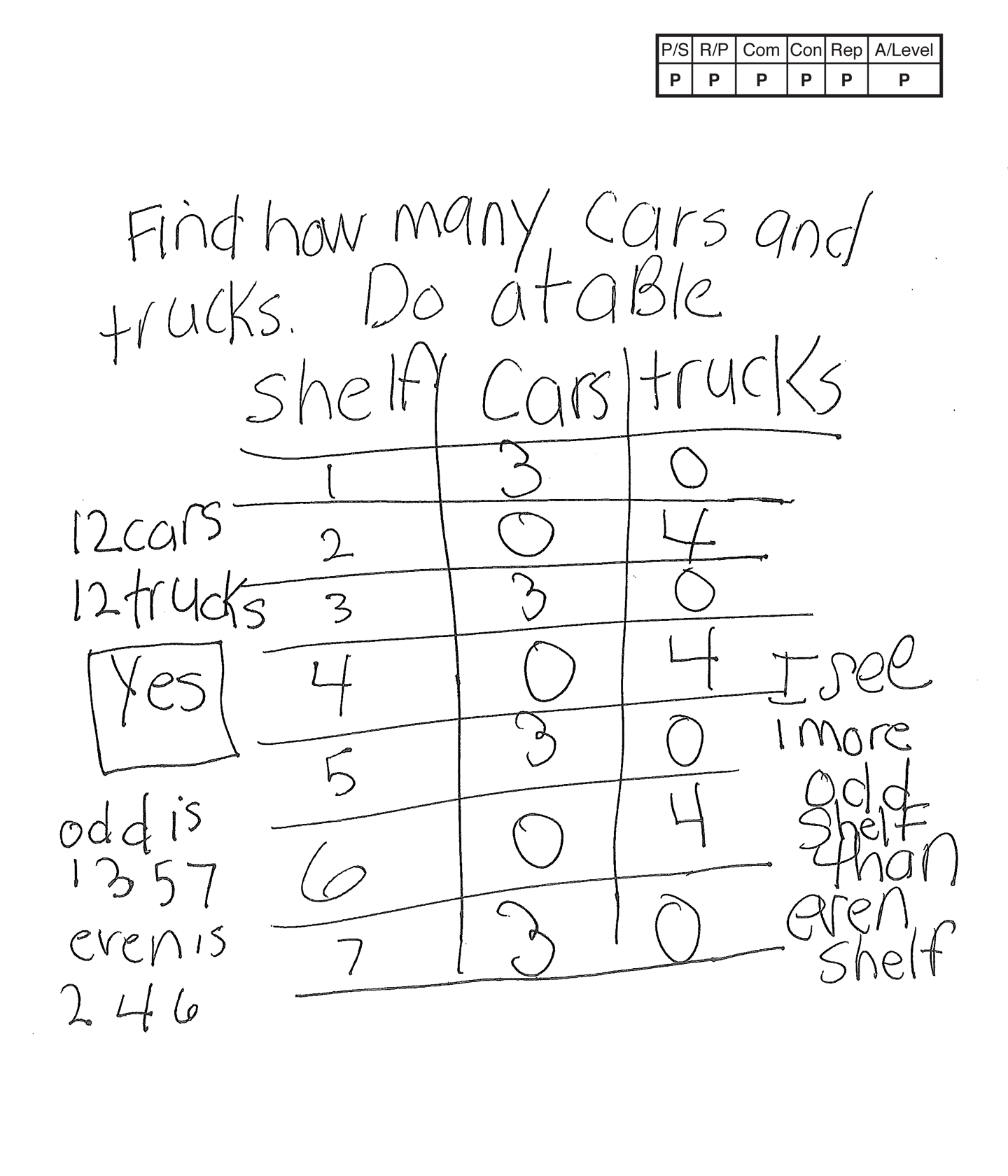This student meets the standard.

### Scoring Rationale

#### Practitioner

The student's table indicating seven shelves with three cars on each odd shelf and four trucks on each even shelf for a total of twelve cars and twelve trucks works to solve the task. The student's answer, "Yes," is correct.

#### Practitioner

The student shows correct reasoning of the underlying concepts of the task. The student understands odd, even, three cars to each odd-numbered shelf, four trucks to each even-numbered shelf, and comparing two totals.

#### Practitioner

The student correctly uses the mathematical terms odd and even from the problem. The student also correctly uses the terms table, more than.

#### Practitioner

The student makes the mathematically relevant connection, "I see 1 more odd shelf than even shelf."

#### Practitioner

The student's table is appropriate to the problem and accurate. All labels are indicated and the data is correct.

#### Practitioner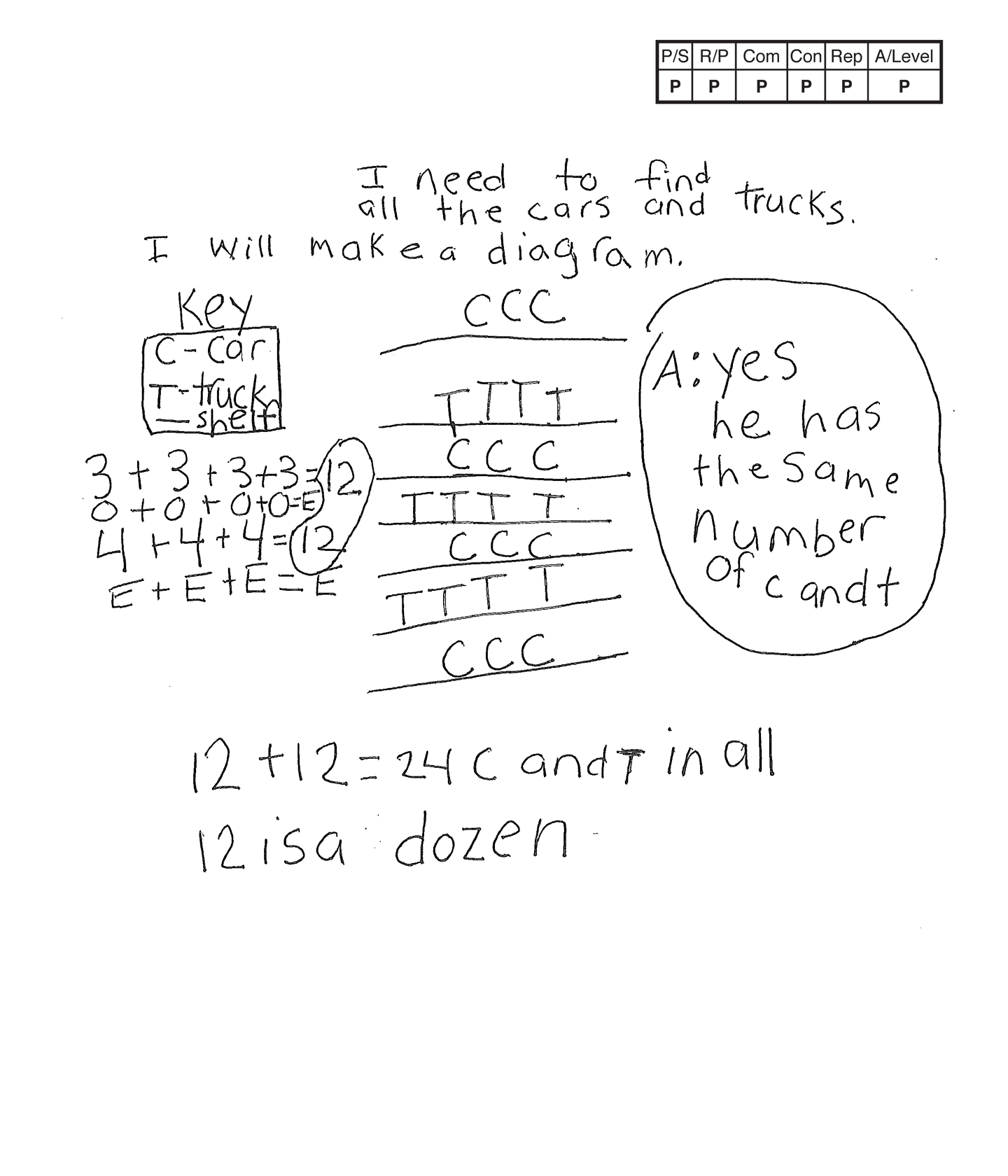This student meets the standard.

### Scoring Rationale

#### Practitioner

The student's diagram indicating seven shelves with three cars on each odd shelf and four trucks on each even shelf for a total of twelve cars and twelve trucks works to solve the task. The student's answer, "Yes," is correct.

#### Practitioner

The student shows correct reasoning of the underlying concepts of the task. The student understands odd, even, three cars to each odd-numbered shelf, four trucks to each even-numbered shelf, and comparing two totals.

#### Practitioner

The student correctly uses the mathematical terms diagram, key, number, dozen.

#### Practitioner

The student makes the mathematically relevant observations, "12 + 12 = 24 C and T in all," and, "12 is a dozen." The student also indicates understanding that Odd + Odd + Odd + Odd = Even, and Even + Even + Even = Even. A key is not provided to define O as Odd and E as Even to support the rules.

#### Practitioner

The student's diagram is appropriate to the task and accurate. A key defines the shelf, cars, and trucks.

#### Practitioner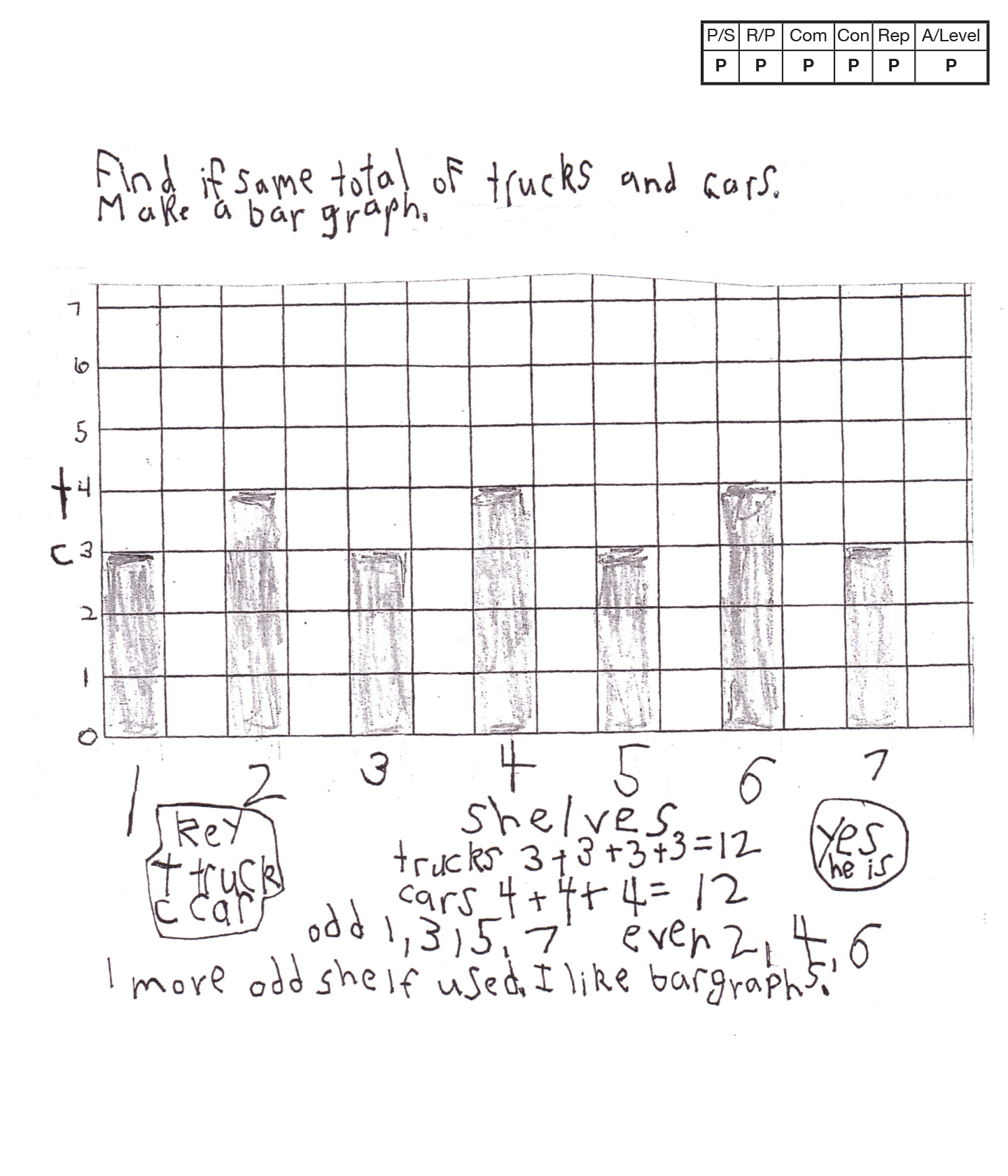,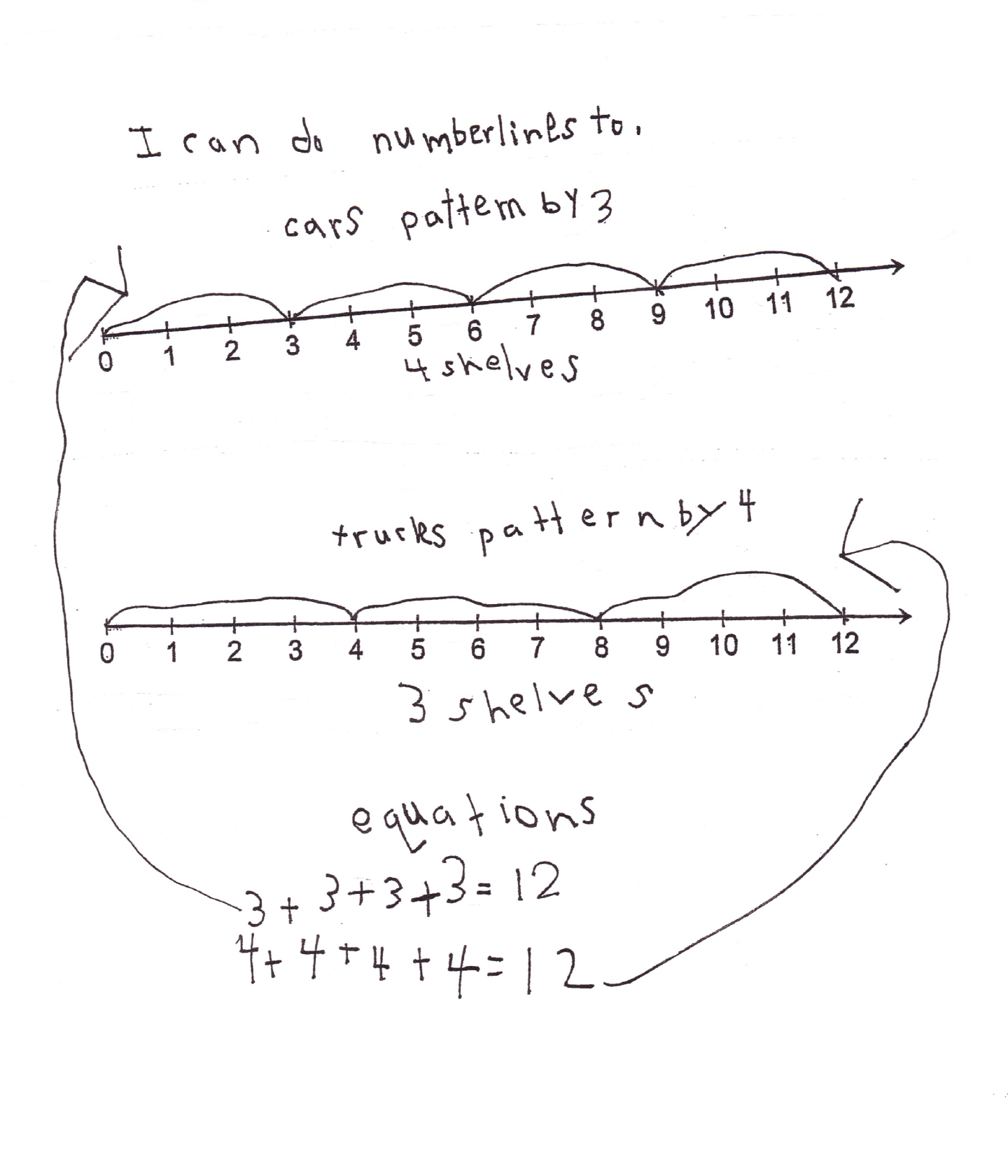This student meets the standard.

### Scoring Rationale

#### Practitioner

The student's strategy of using a bar graph to record the 3 cars on odd shelves and 4 cars on even shelves works to solve the task. The student's answer, "Yes he is" is correct.

#### Practitioner

The student's solution is constructed with adequate mathematical basis. The student demonstrates understanding of odd and even numbers, addition, and comparison.

#### Practitioner

The student correctly uses the mathematical terms total, odd, and even from the task. The student also correctly uses the terms bar graph, key, more, number lines, pattern, and equations.

#### Practitioner

The student makes mathematically relevant connections. The student states, "1 more odd shelf used." The student recreates the task using two number lines to show the cars and trucks on shelves and uses the number to form two equations. The student states, "cars pattern by 3," and "trucks pattern by 4."

#### Practitioner

The student's bar graph is appropriate and accurate. All labels are provided and the entered data is correct. The student's number lines are appropriate and accurate. The numbers and "jumps" are defined.

#### Practitioner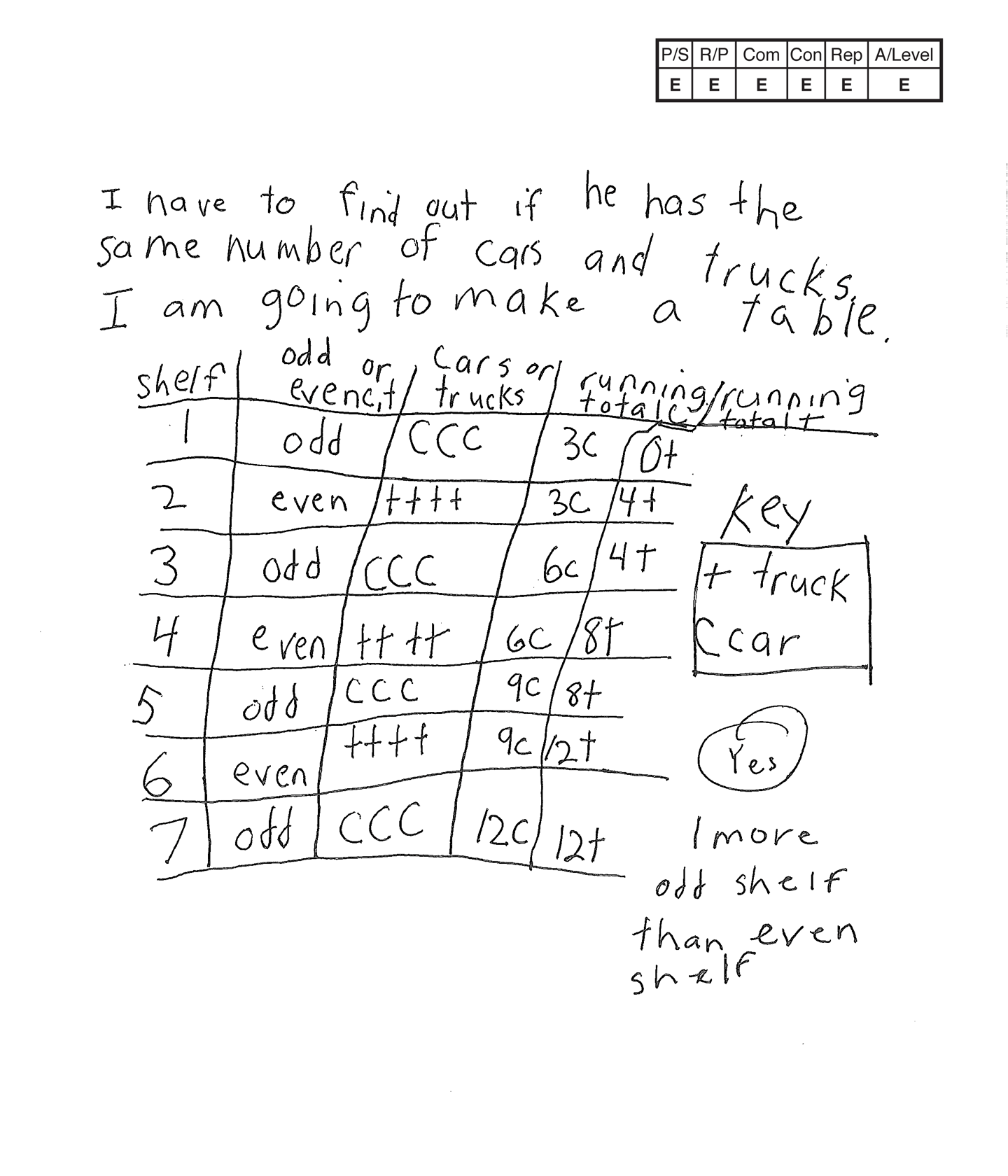,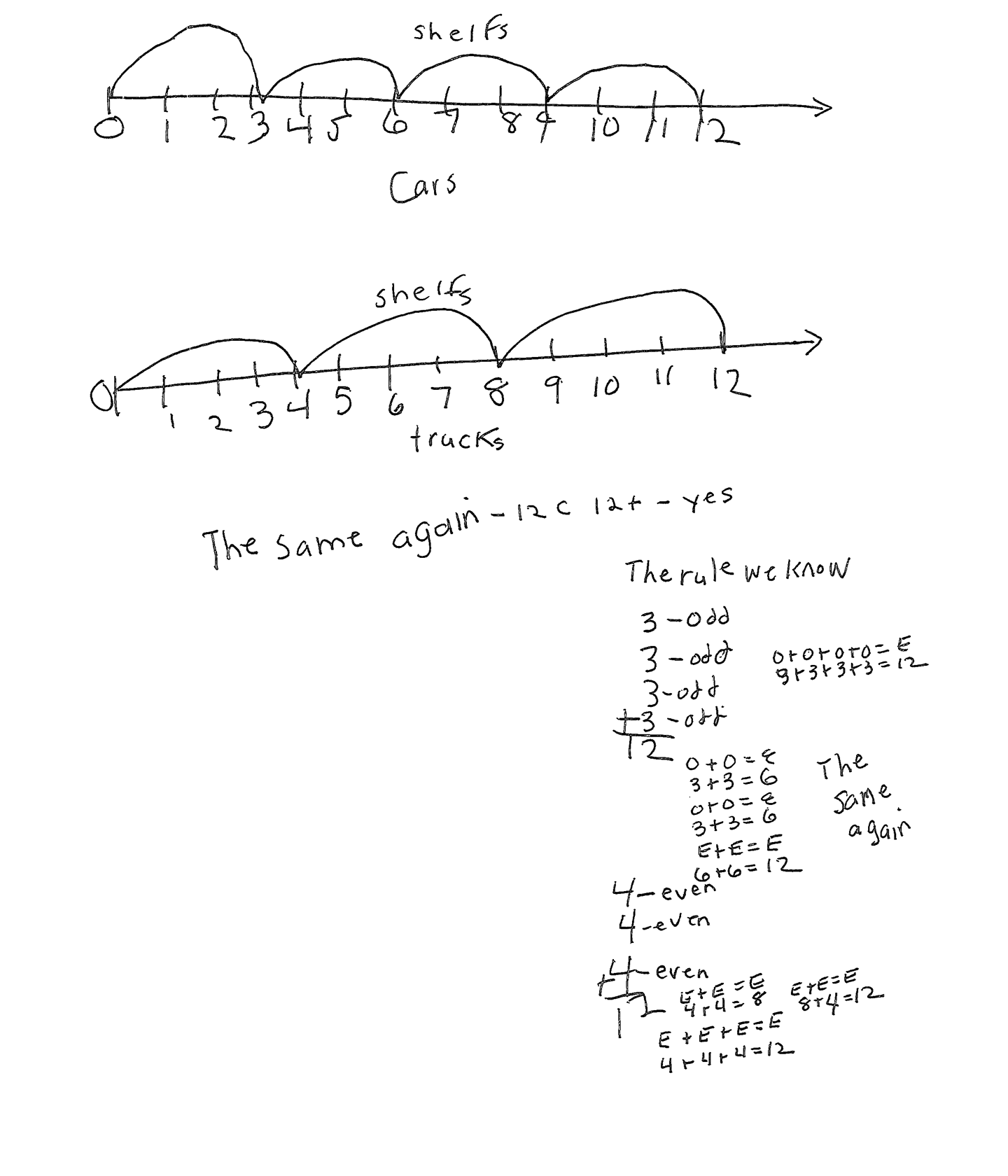,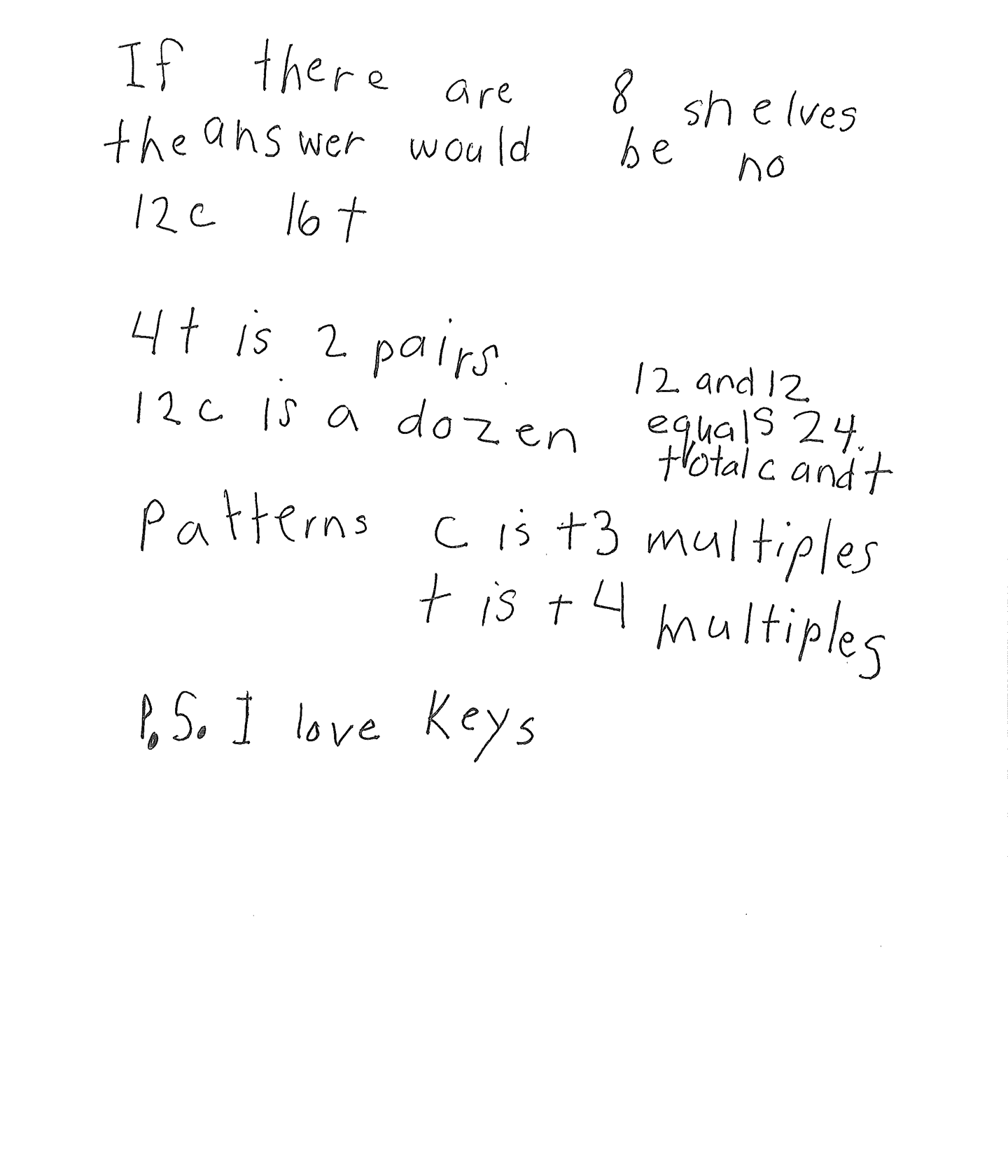This student exceeds the standard.

### Scoring Rationale

#### Expert

The student's table indicating seven shelves with three cars on each odd shelf and four trucks on each even shelf for a total of twelve cars and twelve trucks works to solve the task. The student's answer, "Yes," is correct. The student verifies their answer by making two number lines to indicate twelve cars and twelve trucks. The student also applies the rules used when adding combinations of odd and even numbers.

#### Expert

The student shows correct reasoning of the underlying concepts of the task. The student understands odd, even, three cars to one odd shelf, four trucks to one even shelf, and comparing two totals. The student demonstrates understanding of the resulting sum when adding different combinations of odd and even numbers. The student also verifies their solution is correct and brings the understanding of multiples to their solution.

#### Expert

The student correctly uses the mathematical terms odd, even and number from the task. The student also correctly uses the terms table, key, running total, more than, pairs, dozen, equals, total, patterns, multiples.

#### Expert

The student makes the mathematically relevant Practitioner connections, "1 more odd shelf than even shelf," "If there are eight shelves the answer would be no—12 c, 16 t," "4 t is 2 pairs," "12 c is a dozen," "12 and 12 equals 24, total c and t." The student uses the Expert connections of linking the patterns in the table to multiples, "Patterns c is +3 multiples, t is +4 multiples." The student also uses two number lines to verify that their answer is correct. The student states, "the same again—12 c, 12 t—yes." The student also uses their number line to explain how Odd + Odd + Odd + Odd = Even, and how Even + Even + Even = Even.

#### Expert

The student's table is appropriate to the task and accurate. All labels are indicated and the data is correct. A key defines truck and car. The student's number lines are appropriate and accurate with all necessary labels provided.